# NCERT Solutions for Class 8 Maths Chapter 4 Practical Geometry Ex 4.2

NCERT Solutions for Class 8 Maths Chapter 4 Practical Geometry Exercise 4.2

Ex 4.2 Class 8 Maths Question 1.
LI = 4 cm
IF = 3 cm
TL = 2.5 cm
LF = 4.5 cm
IT = 4 cm
OL = 7.5 cm
GL = 6 cm
GD = 6 cm
LD = 5 cm
OD = 10 cm
(iii) Rhombus BEND
BN = 5.6 cm
DE = 6.5 cm
Solution:
(i) Construction: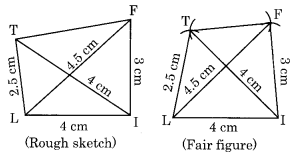Step I: Draw LI = 4 cm.
Step II: Draw an arc with centre I and radius 3 cm.
Step III: Draw another arc with centre L and radius 4.5 cm to meet the former arc at F.
Step IV: Join LF and IF.
Step V: Draw an arc with centre L and radius 2.5 cm.
Step VI: Draw another arc with centre I and radius 4 cm to meet the previous arc at T.
Step VII: Join LT and IT.
Thus LIFT is the required quadrilateral.
(ii) Construction: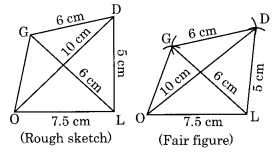Step I: Draw OL = 7.5 cm
Step II: Draw an arc with centre O and radius 10 cm.
Step III: Draw another arc with centre L and radius 5 cm to meet the previous arc at D.
Step IV: Join OD and LD.
Step V: Draw an arc with centre L and D with equal radii of 6 cm to meet each other at G.
Step VI: Join LG and DG.
Thus GOLD is the required quadrilateral.
(iii) Construction: (The diagonals of a rhombus bisect each other at the right angle)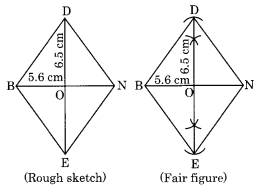Step I: Draw BN = 5.6 cm.
Step II: Draw the right bisector of BN at O.
Step III: Draw two arcs with centre O and radius $$\frac { 1 }{ 2 }$$ × DE, i.e., $$\frac { 1 }{ 2 }$$ × 6.5 = 3.25 cm to meet the right bisector at D and E.
Step IV: Join BE, EN, ND and BD.
Thus, BEND is the required rhombus.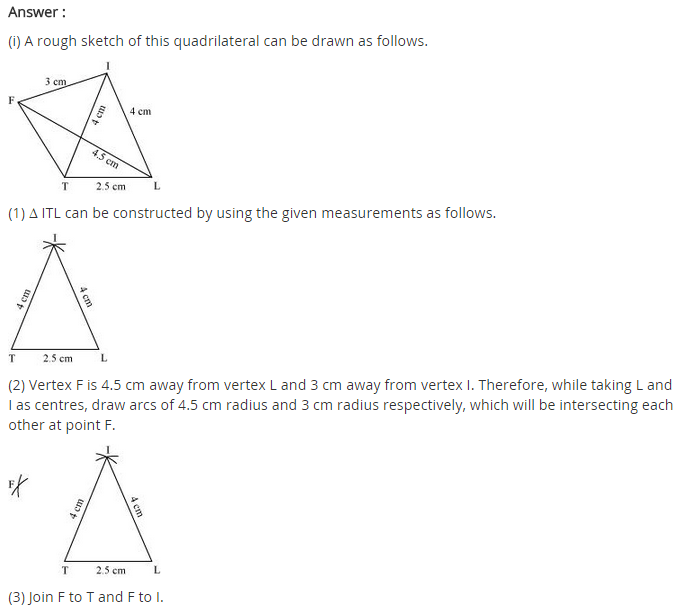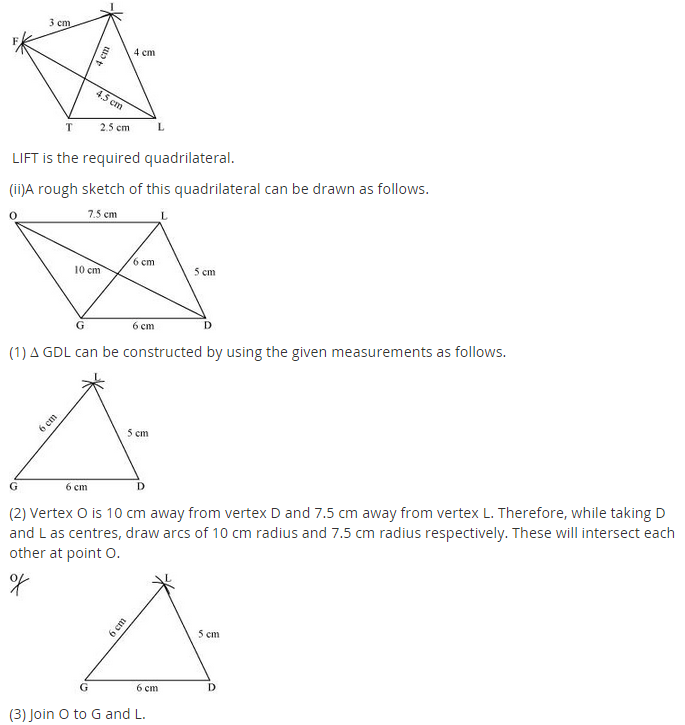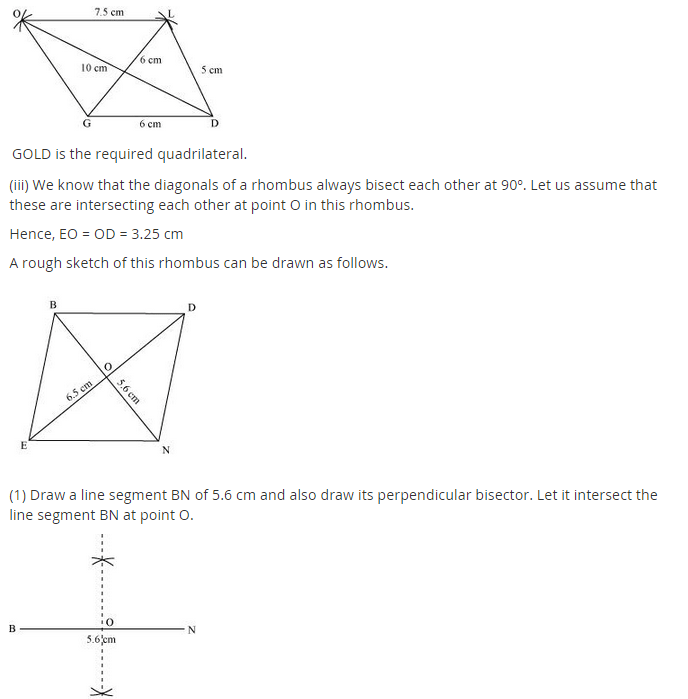+